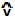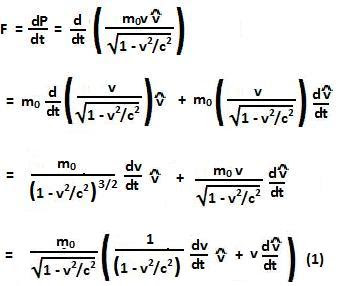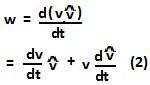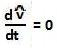## Thursday, January 7, 2010

### Irodov Problem 1.380

a) For this problem let us denote v as the magnitude of the instantaneous velocity of the particle andas the unit vector along the instantaneous direction of motion of the particle. In other words the instantaneous velocity vector of the particle is given by.

From the fundamental laws of dynamics we can write,Similarly, we have,In order for (1) and (2) be parallel there are only two possible ways

1., in which case both vectors get trivially aligned. However, this also means that the particle never changes it direction. In other words, the force has to be aligned in the instantaneous direction of motion of the particle i.e. F is parallel to velocity vector.

2., in which case the magnitude of the velocity of the particle never changes. This can only happen if the force acting on the particle always acts perpendicular to the instantaneous direction of its motion i.e F is perpendicular to the velocity vector.

b) The solution to this part is quite evident from Eqns (1), and (2) considering each of the two cases separately.

Case 1: F is parallel to velocity vectorCase 2 : F is perpendicular to velocity vector